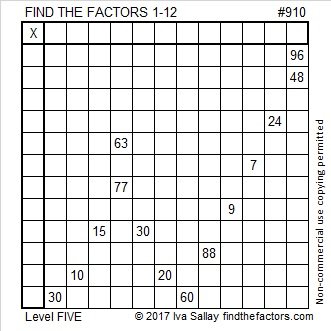# 910 and Level 5

910 is the hypotenuse of four Pythagorean triples:

• 224-882-910, which is 14 times (16-63-65).
• 350-840-910, which is (5-12-13) times 70.
• 462-784-910, which is 14 times (33-56-65)
• 546-728-910, which is (3-4-5) times 182.Print the puzzles or type the solution on this excel file: 12 factors 905-913

910 is 190 in BASE 26, and 910 looks interesting in some other bases, too:

• 4114 in BASE 6, because 4(6³) + 1(6²) + 1(6¹) + 4(6º) = 910
• 1221 in BASE 9, because 1(9³) + 2(9²) + 2(9¹) + 1(9º) = 910
• QQ in BASE 34 (Q is 26 base 10), because 26(34¹) + 26(34º) = 26(34 + 1) = 26(35) = 910
• 26 0 BASE 35, because 26(35) + 0(1) = 26(35) = 910

What are the factors of 910?

• 910 is a composite number.
• Prime factorization: 910 = 2 × 5 × 7 × 13
• The exponents in the prime factorization are 1, 1, 1, and 1. Adding one to each and multiplying we get (1 + 1)(1 + 1)(1 + 1)(1 + 1) = 2 × 2 × 2 × 2 = 16. Therefore 910 has exactly 16 factors.
• Factors of 910: 1, 2, 5, 7, 10, 13, 14, 26, 35, 65, 70, 91, 130, 182, 455, 910
• Factor pairs: 910 = 1 × 910, 2 × 455, 5 × 182, 7 × 130, 10 × 91, 13 × 70, 14 × 65, or 26 × 35
• 910 has no square factors that allow its square root to be simplified. √910 ≈ 30.166206

This site uses Akismet to reduce spam. Learn how your comment data is processed.